Click to Chat

1800-1023-196

+91-120-4616500

CART 0

• 0

MY CART (5)

Use Coupon: CART20 and get 20% off on all online Study Material

ITEM
DETAILS
MRP
DISCOUNT
FINAL PRICE
Total Price: Rs.

There are no items in this cart.
Continue ShoppingElectrolytic Conductance

Table of Content

Specific Conductance or Conductivity

Equivalent Conductance

Molar Conductance

​Measurement of Conductance

Cell Constant

​Effect of Dilution on Equivalent Conductance

Related ResourcesThe conductance is the property of the conductor (metallic as well as electrolytic) which facilitates the flow of electricity through it. It is equal to the reciprocal of resistance i.e.,

Units of conductance :  .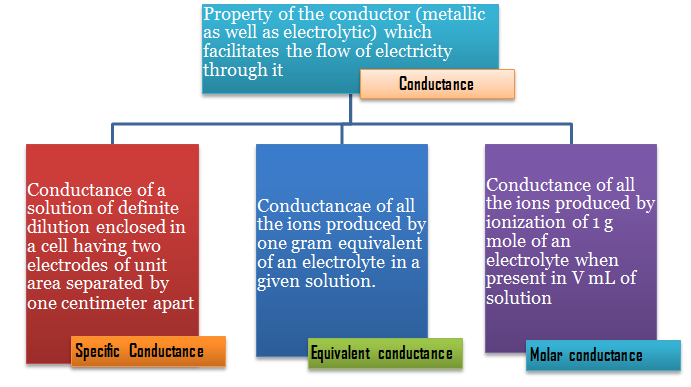Specific Conductance or Conductivity

The resistance of any conductor varies directly as its length (l) and inversely as its cross-sectional area (A), i.e.,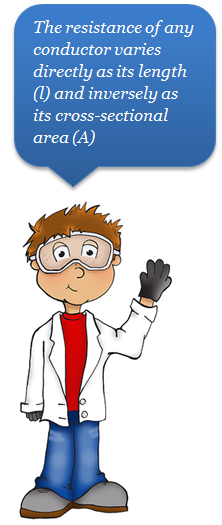Mathematically

Where ρ is called the specific resistance.

l/A is known as cell constant.

If l = 1 cm and A = 1 cm2, then

R = ρ

The specific resistance is, thus defined as the resistance of one centimeter cube of a conductor.

The reciprocal of specific resistance is termed the specific conductance or it is the conductance of one centimeter cube of a conductor.

It is denoted by the symbo  (kappa)l .

Thus,

Where   kappa →  the specific conductance

Specific conductance is also called conductivity.

Further,

or  Specific conductance = Conductance × cell constant

In the case of electrolytic solutions, the specific conductance is defined as the conductance of a solution of definite dilution enclosed in a cell having two electrodes of unit area separated by one centimeter apart.

Unit of specific conductance:

Equivalent Conductance

One of the factors on which the conductance of an electrolytic solution depends is the concentration of the solution.

In order to obtain comparable results for different electrolytes, it is necessary to take equivalent conductance's.

Equivalent  conductance is defined as the conductance of all the ions produced by one gram equivalent of an electrolyte in a given solution.

It is denoted by A.

To understand the manning of equivalent conductance, imagine a rectangular trough with two opposite sides made of metallic conductor (acting as electrodes) exactly 1 cm apart, If 1 cm3 (1 mL) solution containing 1 gram equivalent of an electrolyte is places in this container is measured.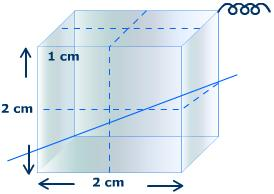According to definitions,

Conductance = Specific conductance (K)  = Equivalent conductance ()

If the solution is diluted to say (9 cm3) (9 mL), the conductance of the solution will be the same but specific conductance becomes 1/9th as it contains nine cubes.

The conductance is also equal to the equivalent because the solution still has 1 g equivalent of the electrolyte.

Equivalent conductance () = 9 × k

In general,

= k × V

where V is the volume in mL containing 1 g equivalent of the electrolyte.

In case, if the concentration of the solution is c gram equivalent per litre, then the volume containing 1 g equivalent of the electrolyte will be 1000/e.

So equivalent conductance

= k ×  1000/c

= k ×  1000/N

where N = normality

Unit of equivalent conductance:

Molar Conductance

The molar conductance is defined as the conductance of all the ions produced by ionization of 1 g mole of an electrolyte when present in V mL of solution. It is denoted by .

Molar conductance     μ = k ×V

where V is the volume in mL containing 1 g mole of the electrolyte. If c is the concentration of the solution in g mole per litre, then

μ  = k ×  1000/c

Units of molar conductance:

Equivalent conductance =  (Molar conductance)/n

where

n =  (Molecular mass)/(Equivalent mass)​
Measurement of Conductance

It is now known to us that when the solution of an electrolyte is taken between two parallel electrodes of cross-sectional area 'a' and 'l' cm apart, then the specific conductance, k, should be:

Thus, knowing the values of R, l and A, the specific conductance can be measured.

The resistance of the solution between two parallel electrodes is determined by using Wheatstone bridge method.

The diagram of the apparatus is shown in AB is a uniform wire and X is a sliding contact which moves over it.

C is the conductivity cell containing the solution of the electrolyte and S represents the source of alternating current.

R is the resistance box and T is a headphone to detect the flow of current.

A suitable resistance is taken out from the resistance box and the sliding contact X is moves on the wire to search a point of minimum sound in the headphone. At this point, the bridge is balanced.

(Resistance of solution)/(Resistance from resistance box)  =(Resistance XB)/(Resistance XA)  =(Length XB)/(Length XA)

Resistance of solution can be used because it produce two complications.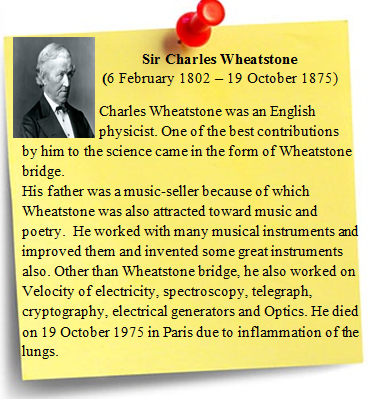Change in the concentration of the solution occurs due to electrolysis which will change the resistance.

Polarisation at the electrodes sets in which also charges the resistance.

An alternating current (A.C.) is used to overcome the above complications.

The solution whose conductance is to be measured is taken in a special type of cell known as conductivity as conductivity cell.

The electrodes consist of platinum discs coated with finely divided platinum black and welded to platinum wires fused in two glass tubes.

The glass tubes contain mercury and are finely fixed in the cover of cells. Contact with the platinum is made by dipping the copper wires of the circuit in the mercury contained in the tubes.

As the conductivity changes with temperature, the cell is usually placed in a constant temperature bath during the experiment.

Cells with along paths are used for concentrated solution and cells with sort paths and large electrodes are used for dilute solutions.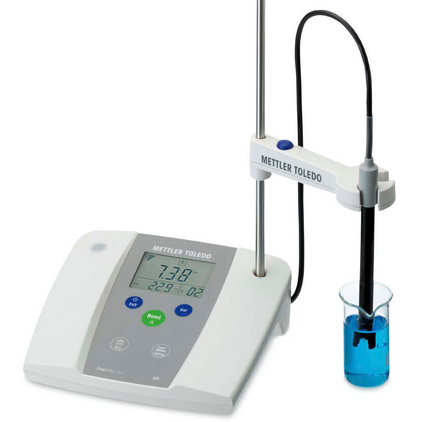Cell Constant

Since the electrodes are not exactly 1 unit apart and may not possess a surface area of 1 square unit, the measured resistance does not give the specific conductance of the solution. Actual measurements of l and a being inconvenient, an indirect method is employed to determine the value of  which is a constant quantity for a particular cell and is known as cell constant.

We know that

(Specific conductance)/Conductance=l/A= cell constant

The resistance of cell, i.e., conductance is measured when filled with a standard solution (say N/10 KCl solution) at a given temperature.

The standard values of specific conductance of KCl solutions of various concentrations at different temperature are known.

Thus, the cell constant is calculated by using the above equation. The sane cell constant applies to a measurement with any other solution.

The determination of specific conductance of an electrolytic solution, thus, consists of two steps:

Determination of cell constant by using a standard KCl solution of known concentration in the conductivity cell.

Determination of resistance of he given solution using the same cell. The reciprocal of this gives the value of conductance.

Multiplication of conductance and cell constant gives the value of specific conductance of the solution.

In order to determine equivalent conductance or molar conductance, the concentration of the experimental solution should be known.

In conductance measurements, the solutions are always prepared in conductivity water which has no conductance due to dissolved impurities.

It is prepared by distilling a number of times the distilled water to which a little KMnO4 and KOH have been added in a hard glass distillation assembly.

Such water has very low conductance of the order of 4.3 × 10-8 ohm-1. For ordinary purposes, double distilled water may be used.

​Effect of Dilution on Equivalent Conductance

The value of equivalent conductance increases with dilution.

This is due to the fact that degree of ionization increases with dilution thereby increasing the total number of ions in solution.

Solution which contains large number of ions compared to another solution of the same concentration at the same temperature has more conductance and is said to be stronger electrolyte.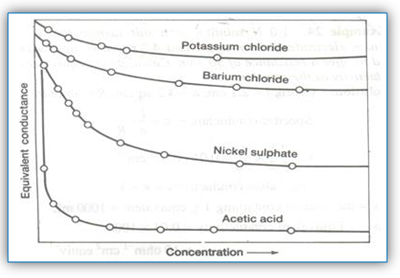The one which has relatively small number of ions is called a weak electrolyte. The number of ions from an electrolyte depends on the degree of dissociation. The curve shows the variation of the equivalent conductance of some electrolytes with dilution. It shows that electrolytes behave in two ways o dilution.

Electrolytes like KCl have high value of conductance even at low concentration and there is no rapid increase in their equivalent conductance on dilution. Such electrolytes are termed strong electrolytes. In the case of strong electrolytes, there is a tendency for equivalent conductance to approach a limiting value when the concentration approaches zero. When the whole of the electrolyte has ionized, further addition of the water does not bring any change in the value of equivalent conductance. This stage is called infinite dilution. The equivalent conductance has a limiting value oat infinite dilution and is represented by /\∞ .

Electrolytes like acetic acid have a low value at high concentration and there is a rapid increase in the value of equivalent conductance with dilution. Such electrolytes are termed weak electrolytes. There is no indication that a limiting value of equivalent conductance can be attained even when the concentration approaches zero. Thus, graphically, /\∞  of weak electrolytes cannot be obtained.

It is thus concluded that equivalent conductance of electrolytes whether strong or weak increases with dilution and reaches to a maximum or limiting value which is termed  /\∞ (equivalent conductance at infinite dilution.) /\∞  in the case of strong electrolytes cannot be obtained by extrapolation of the graph of equivalent conductance to zero concentration but in the case of weak electrolytes it cannot be obtained accurately. An indirect method for obtaining  /\∞ for weak electrolyte has been given by Kohlrusch.Question 1:

Unit of molar conductivity is

a. ohm cm-1 mol-l

b. ohm-1 mol

c. ohm-1 cm-1 mol-l

d. ohm  mol-l

Question 2:

The reciprocal of resistivity is known as

a. molar conductance

b. specific conductance

c. equivalent conductance

d. permittivity

Question 3:

is the unit of

a. molar conductance

b. specific conductance

c. conductivity

d. resistivity

Question 4:

The value of equivalent conductance

a.decreases with pressure.

b. increases with pressure.

c. decreases with dilution.

d. increases with dilution.Q.1

Q.2

Q.3

Q.4

a

b

a

d

Related Resources

Refer to the  Syllabus of IIT JEE

Look into the Sample Papers for an idea about type of questions asked in IIT JEE.

You can also refer to Electrochemical Series

To read more, Buy study materials of Electrochemistry comprising study notes, revision notes, video lectures, previous year solved questions etc. Also browse for more study materials on Chemistry here.### Course Features

• 731 Video Lectures
• Revision Notes
• Previous Year Papers
• Mind Map
• Study Planner
• NCERT Solutions
• Discussion Forum
• Test paper with Video Solution